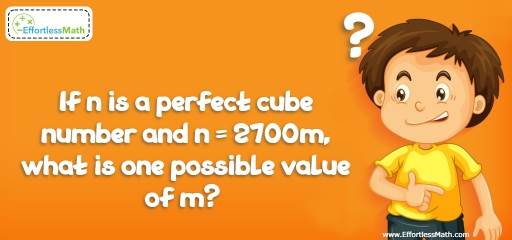# Number Properties Puzzle – Challenge 20

Looking for maths puzzles with answers? If so, you are in the right place. The solution is beneficial when trying to solve a hard puzzle like this one.## Challenge:

If n is a perfect cube number and n = 2700m, what is one possible value of m?

### The Absolute Best Book to Challenge Your Smart Student!

Factorize 2700:
$$2700 = 2^2 × 3^3 × 5^2$$
The product of two perfect cube numbers is another perfect cube number. For example 8 and 27 are perfect cube numbers. The product of 8 and 27 is 216, which is another perfect cube number. (8 × 27 = 216 and $$6^3 = 216$$)
To make 2700m a perfect cube number we need the product of 5 and 2.
$$2700 = 2^2 × 3^3 × 5^2$$
$$2700 × 10 = 2^3 × 3^3 × 5^3$$, therefore, m = 10

The Absolute Best Books to Ace Algebra

### What people say about "Number Properties Puzzle – Challenge 20 - Effortless Math: We Help Students Learn to LOVE Mathematics"?

No one replied yet.

X
45% OFF

Limited time only!

Save Over 45%

SAVE $40 It was$89.99 now it is \$49.99